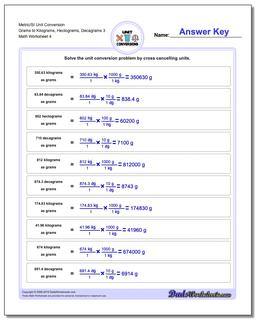# Math Worksheets: Metric SI Unit Conversions: Metric SI Unit Conversions: Metric/SI Unit Conversion Grams to Kilograms, Hectograms, Decagrams 3 (Fourth Worksheet)## Metric/SI Unit Conversion Grams to Kilograms, Hectograms, Decagrams 3 (Fourth Worksheet)

PropertyValue
DescriptionMetric/SI Unit Conversion Grams to Kilograms, Hectograms, Decagrams 3: The conversion worksheets in this section practice converting metric/SI unit mass (gram base units) to other metric units. (Fourth Worksheet)
Resource TypeWorksheet